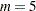# Getting Started: MIANALYZE Procedure

The Fitness data described in the REG procedure are measurements of 31 individuals in a physical fitness course. See Chapter 76, The REG Procedure, for more information. The Fitness1 data set is constructed from the Fitness data set and contains three variables: Oxygen, RunTime, and RunPulse. Some values have been set to missing, and the resulting data set has an arbitrary pattern of missingness in these three variables.

```*----------------- Data on Physical Fitness -----------------*
| These measurements were made on men involved in a physical |
| fitness course at N.C. State University.                   |
| Only selected variables of                                 |
| Oxygen (oxygen intake, ml per kg body weight per minute),  |
| Runtime (time to run 1.5 miles in minutes), and            |
| RunPulse (heart rate while running) are used.              |
| Certain values were changed to missing for the analysis.   |
*------------------------------------------------------------*;
data Fitness1;
input Oxygen RunTime RunPulse @@;
datalines;
44.609  11.37  178     45.313  10.07  185
54.297   8.65  156     59.571    .      .
49.874   9.22    .     44.811  11.63  176
.     11.95  176          .  10.85    .
39.442  13.08  174     60.055   8.63  170
50.541    .      .     37.388  14.03  186
44.754  11.12  176     47.273    .      .
51.855  10.33  166     49.156   8.95  180
40.836  10.95  168     46.672  10.00    .
46.774  10.25    .     50.388  10.08  168
39.407  12.63  174     46.080  11.17  156
45.441   9.63  164       .      8.92    .
45.118  11.08    .     39.203  12.88  168
45.790  10.47  186     50.545   9.93  148
48.673   9.40  186     47.920  11.50  170
47.467  10.50  170
;
```

Suppose that the data are multivariate normally distributed and that the missing data are missing at random (see the "Statistical Assumptions for Multiple Imputation" section in the chapter "The MI Procedure" for a description of these assumptions). The following statements use the MI procedure to impute missing values for the Fitness1 data set:

```proc mi data=Fitness1 seed=3237851 noprint out=outmi;
var Oxygen RunTime RunPulse;
run;
```

The MI procedure creates imputed data sets, which are stored in the outmi data set. A variable named _Imputation_ indicates the imputation numbers. Based onimputations,different sets of the point and variance estimates for a parameter can be computed. In this example,is the default.

The following statements generate regression coefficients for each of the five imputed data sets:

```proc reg data=outmi outest=outreg covout noprint;
model Oxygen= RunTime RunPulse;
by _Imputation_;
run;
```

The following statements display (in Figure 57.1) output parameter estimates and covariance matrices from PROC REG for the first two imputed data sets:

```proc print data=outreg(obs=8);
var _Imputation_ _Type_ _Name_
Intercept RunTime RunPulse;
title 'Parameter Estimates from Imputed Data Sets';
run;
```

Figure 57.1 Parameter Estimates
 Parameter Estimates from Imputed Data Sets

Obs _Imputation_ _TYPE_ _NAME_ Intercept RunTime RunPulse
1 1 PARMS   86.544 -2.82231 -0.05873
2 1 COV Intercept 100.145 -0.53519 -0.55077
3 1 COV RunTime -0.535 0.10774 -0.00345
4 1 COV RunPulse -0.551 -0.00345 0.00343
5 2 PARMS   83.021 -3.00023 -0.02491
6 2 COV Intercept 79.032 -0.66765 -0.41918
7 2 COV RunTime -0.668 0.11456 -0.00313
8 2 COV RunPulse -0.419 -0.00313 0.00264

The following statements combine the five sets of regression coefficients:

```proc mianalyze data=outreg;
modeleffects Intercept RunTime RunPulse;
run;
```

The "Model Information" table in Figure 57.2 lists the input data set(s) and the number of imputations.

Figure 57.2 Model Information Table
The MIANALYZE Procedure

Model Information
Data Set WORK.OUTREG
Number of Imputations 5

The "Variance Information" table in Figure 57.3 displays the between-imputation, within-imputation, and total variances for combining complete-data inferences. It also displays the degrees of freedom for the total variance, the relative increase in variance due to missing values, the fraction of missing information, and the relative efficiency for each parameter estimate.

Figure 57.3 Variance Information Table
Variance Information
Parameter Variance DF Relative
Increase
in Variance
Fraction
Missing
Information
Relative
Efficiency
Between Within Total
Intercept 45.529229 76.543614 131.178689 23.059 0.713777 0.461277 0.915537
RunTime 0.019390 0.106220 0.129487 123.88 0.219051 0.192620 0.962905
RunPulse 0.001007 0.002537 0.003746 38.419 0.476384 0.355376 0.933641

The "Parameter Estimates" table in Figure 57.4 displays a combined estimate and standard error for each regression coefficient (parameter). Inferences are based on t distributions. The table displays a 95% confidence interval and a t test with the associated p-value for the hypothesis that the parameter is equal to the value specified with the THETA0= option (in this case, zero by default). The minimum and maximum parameter estimates from the imputed data sets are also displayed.

Figure 57.4 Parameter Estimates
Parameter Estimates
Parameter Estimate Std Error 95% Confidence Limits DF Minimum Maximum Theta0 t for H0:
Parameter=Theta0
Pr > |t|
Intercept 90.837440 11.453327 67.14779 114.5271 23.059 83.020730 100.839807 0 7.93 <.0001
RunTime -3.032870 0.359844 -3.74511 -2.3206 123.88 -3.204426 -2.822311 0 -8.43 <.0001
RunPulse -0.068578 0.061204 -0.19243 0.0553 38.419 -0.112840 -0.024910 0 -1.12 0.2695